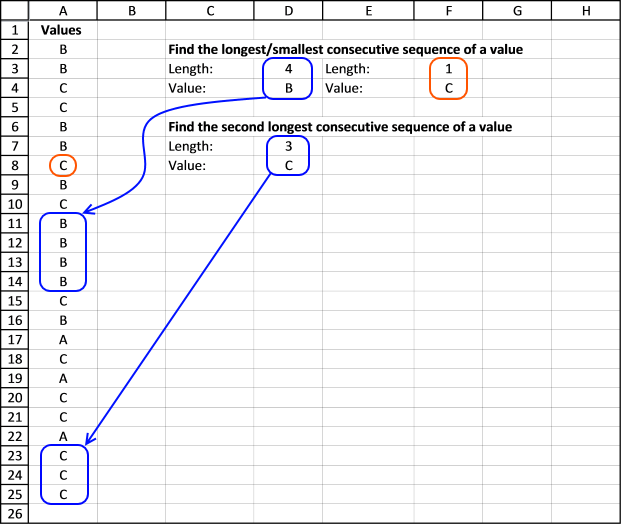Author: Oscar Cronquist Article last updated on January 20, 2018

The array formula in cell D3 returns the the length of longest consecutive sequence of a value in column A.  Cell D4 returns the value of the longest consecutive sequence in column A.

Cell F3 returns the the length of the shortest consecutive sequence of a value in column A and cell F4 returns the value of the smallest consecutive sequence in column A.Length of longest consecutive sequence of a value

Array formula in cell D3:

=MAX(FREQUENCY(IF(\$A\$2:\$A\$25<>\$A\$3:\$A\$26, "", MATCH(ROW(\$A\$2:\$A\$25), ROW(\$A\$2:\$A\$25))), IF(\$A\$2:\$A\$25<>\$A\$3:\$A\$25, MATCH(ROW(\$A\$2:\$A\$25), ROW(\$A\$2:\$A\$25)) ,""))+1)

How to enter an array formula

1. Select cell
2. Copy/Paste the formula to the formula bar
3. Press and hold CTRL + SHIFT simultaneously
4. Press Enter once
5. Release all keys

Value of the longest consecutive sequence

Array formula in cell D4:

=INDEX(\$A\$2:\$A\$25, SMALL(IF(\$A\$2:\$A\$25<>\$A\$3:\$A\$26, MATCH(ROW(\$A\$2:\$A\$25), ROW(\$A\$2:\$A\$25)), ""), MATCH(MAX(FREQUENCY(IF(\$A\$2:\$A\$25<>\$A\$3:\$A\$26, "", MATCH(ROW(\$A\$2:\$A\$25), ROW(\$A\$2:\$A\$25))), IF(\$A\$2:\$A\$25<>\$A\$3:\$A\$26, MATCH(ROW(\$A\$2:\$A\$25), ROW(\$A\$2:\$A\$25)), ""))+1), FREQUENCY(IF(\$A\$2:\$A\$25<>\$A\$3:\$A\$26, "", MATCH(ROW(\$A\$2:\$A\$25), ROW(\$A\$2:\$A\$25))), IF(\$A\$2:\$A\$25<>\$A\$3:\$A\$26, MATCH(ROW(\$A\$2:\$A\$25), ROW(\$A\$2:\$A\$25)), ""))+1, 0)))

I wish I could present a smaller formula.

Length of shortest consecutive sequence of a value

Array formula in cell F3:

=MIN(FREQUENCY(IF(\$A\$2:\$A\$25<>\$A\$3:\$A\$26, "", MATCH(ROW(\$A\$2:\$A\$25), ROW(\$A\$2:\$A\$25))), IF(\$A\$2:\$A\$25<>\$A\$3:\$A\$25, MATCH(ROW(\$A\$2:\$A\$25), ROW(\$A\$2:\$A\$25)) ,""))+1)

Value of the shortest consecutive sequence

Array formula in cell F4:

=INDEX(\$A\$2:\$A\$25, SMALL(IF(\$A\$2:\$A\$25<>\$A\$3:\$A\$26, MATCH(ROW(\$A\$2:\$A\$25), ROW(\$A\$2:\$A\$25)), ""), MATCH(MIN(FREQUENCY(IF(\$A\$2:\$A\$25<>\$A\$3:\$A\$26, "", MATCH(ROW(\$A\$2:\$A\$25), ROW(\$A\$2:\$A\$25))), IF(\$A\$2:\$A\$25<>\$A\$3:\$A\$26, MATCH(ROW(\$A\$2:\$A\$25), ROW(\$A\$2:\$A\$25)), ""))+1), FREQUENCY(IF(\$A\$2:\$A\$25<>\$A\$3:\$A\$26, "", MATCH(ROW(\$A\$2:\$A\$25), ROW(\$A\$2:\$A\$25))), IF(\$A\$2:\$A\$25<>\$A\$3:\$A\$26, MATCH(ROW(\$A\$2:\$A\$25), ROW(\$A\$2:\$A\$25)), ""))+1, 0)))

Length of second longest consecutive sequence of a value

Array formula in cell D7:

=LARGE(FREQUENCY(IF(\$A\$2:\$A\$25<>\$A\$3:\$A\$26, "", MATCH(ROW(\$A\$2:\$A\$25), ROW(\$A\$2:\$A\$25))), IF(\$A\$2:\$A\$25<>\$A\$3:\$A\$25, MATCH(ROW(\$A\$2:\$A\$25), ROW(\$A\$2:\$A\$25)), ""))+1, 2)

Value of the second longest consecutive sequence

Array formula in cell D8:

=INDEX(\$A\$2:\$A\$25, SMALL(IF(\$A\$2:\$A\$25<>\$A\$3:\$A\$26, MATCH(ROW(\$A\$2:\$A\$25), ROW(\$A\$2:\$A\$25)), ""), MATCH(LARGE(FREQUENCY(IF(\$A\$2:\$A\$25<>\$A\$3:\$A\$26, "", MATCH(ROW(\$A\$2:\$A\$25), ROW(\$A\$2:\$A\$25))), IF(\$A\$2:\$A\$25<>\$A\$3:\$A\$26, MATCH(ROW(\$A\$2:\$A\$25), ROW(\$A\$2:\$A\$25)), ""))+1, 2), FREQUENCY(IF(\$A\$2:\$A\$25<>\$A\$3:\$A\$26, "", MATCH(ROW(\$A\$2:\$A\$25), ROW(\$A\$2:\$A\$25))), IF(\$A\$2:\$A\$25<>\$A\$3:\$A\$26, MATCH(ROW(\$A\$2:\$A\$25), ROW(\$A\$2:\$A\$25)), ""))+1, 0)))

If you are interested in learning and constructing array formulas, check out my Advanced excel course.

Longest consecutive sequence.xlsx

Functions in this post

FREQUENCY(data_array, bins_array)
Calculates how often values occur within a range of values and then returns a vertical array of numbers having one more element than the bins_array

IF(logical_test;[value_if:true];[value_if_false])
Checks whether a condition is met, and returns one value if TRUE, and another value if FALSE.

SMALL(array,k)
Returns the k-th smallest number in this data set.

ROW(reference)
Returns the rownumber of a reference.

MATCH(lookup_value, lookup_array, [match_type]
Returns the relative position of an item in an array that matches a specified value.